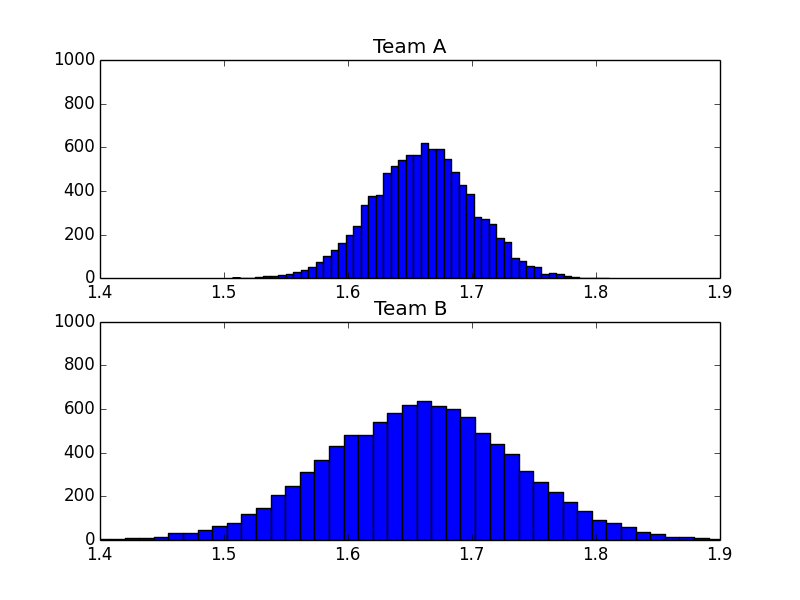# [PYTHON] Monte Carlo method

The Monte Carlo method is a method of converting the integral of a function into the sum of sampled points so as to reproduce a given function.

[Wikipedia](http://en.wikipedia.org/wiki/%E3%83%A2%E3%83%B3%E3%83%86%E3%82%AB%E3%83%AB%E3%83 This depends on the quality of random number generation, as described in% AD% E6% B3% 95).

### The principle of Monte Carlo method

The principle of numerical calculation by the Monte Carlo method is that in the questionnaire, a large number of samples are extracted from the department to which many people belong, and a small number of samples are extracted from the department to which a small number of people belong. It's similar to the idea that it can be reflected in a questionnaire. For example, to calculate pi, generate a set of random numbers in the range of 0 to 1, see it as a point on the xy coordinates, and determine whether it is in the first quadrant of the unit circle or not from that ratio. ..

For random variables that take consecutive values, the probability distribution is represented by the probability density function f (x). At this time, the mean value u and the variance σ ^ 2 are as follows.

\mu = \int{xf(x)dx} \\
\sigma^2 = \int{(x - \mu)}^2f(x)dx


### Actually try with code

There were two sports teams A and B. The two sports teams scored exactly the same, but with different variances. This is expressed by Monte Carlo simulation.

from pylab import *
from scipy.stats import *
import matplotlib.pyplot as plt

runs = 10000

#Results of each team
teamperfA = [0.9,0.8,0,9] #Team A score
teamperfB = [0.9,0.8,0.9] #Team B score

#Dispersion of results by team
teamvarianceA = [0.03, 0.04, 0.02]
teamvarianceB = [0.05, 0.08, 0.09] #Team B has higher variance

weights = [1.0, 0.95, 0.8]

def result(perf,variance,weights):
res = 0.0
for i in range(len(perf)-1):
res += perf[i] * weights[i] * norm(1,variance[i]).rvs()

return res

resultsA = zeros(shape=(runs,), dtype=float)
resultsB = zeros(shape=(runs,), dtype=float)

for i in range(runs):
resultsA[i] = result (teamperfA,teamvarianceA,weights)
resultsB[i] = result (teamperfB,teamvarianceB,weights)

subplot(211)
width = 2
height=runs

title('Team A')
hist(resultsA, bins=50)
axis([1.4,1.9,0,height/10])

subplot(212)
title('Team B')
hist(resultsB, bins=50)

axis([1.4,1.9,0,height/10])

show()
plt.savefig("image.png ")## reference

Python matplotlib, Monte Carlo simulation, and basic statistics http://softwaredevelopmentperestroika.wordpress.com/2013/12/06/python-matplotlib-monte-carlo-simulation-and-basic-statistics/